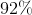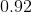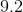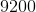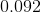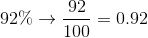# Algebra 1 : How to find decimal equivalent to a percentage

## Example Questions

### Example Question #11 : How To Find Decimal Equivalent To A Percentage

Convert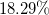to a decimal.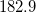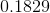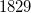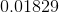Explanation:

To convert a percentage to a decimal, we simply move the decimal point two places to the left.

Alternatively, to convert from a decimal to a percentage, we would move the decimal place two decimal places the right.

But in this case, we are going from percentage to a decimal, so starting with, moving the decimal places two places to the left would leave us with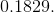Another way to solve is to divide the percentage by one hundred: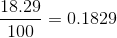### Example Question #12 : How To Find Decimal Equivalent To A Percentage

Find the decimal equivalent of the following percent: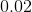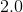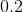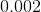Explanation:

In order to find the decimal equivalent for a percent, all we have to do is divide our percent by 100. We can also move the decimal point to the left two spaces.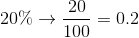### Example Question #13 : How To Find Decimal Equivalent To A Percentage

Find the decimal equivalent for the following percent: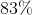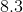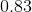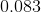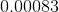Explanation:

In order to find the decimal equivalent for a percent, all we have to do is divide our percent by 100. We can also move the decimal point to the left two spaces.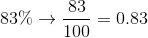### Example Question #14 : How To Find Decimal Equivalent To A Percentage

Find the decimal equivalent of the following percent: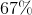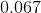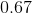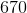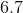Explanation:

In order to find the decimal equivalent for a percent, all we have to do is divide our percent by 100. We can also move the decimal point to the left two spaces.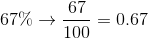### Example Question #15 : How To Find Decimal Equivalent To A Percentage

Find the decimal equivalent of the following percent: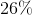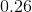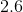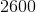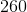Explanation:

In order to find the decimal equivalent for a percent, all we have to do is divide our percent by 100. We can also move the decimal point to the left two spaces.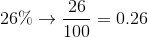### Example Question #16 : How To Find Decimal Equivalent To A Percentage

Find the decimal equivalent of the following percent: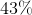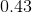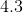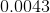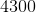Explanation:

In order to find the decimal equivalent for a percent, all we have to do is divide our percent by 100. We can also move the decimal point to the left two spaces.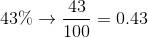### Example Question #17 : How To Find Decimal Equivalent To A Percentage

Find the decimal equivalent of the following percent: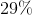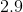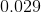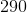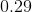Explanation:

In order to find the decimal equivalent for a percent, all we have to do is divide our percent by 100. We can also move the decimal point to the left two spaces.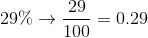### Example Question #18 : How To Find Decimal Equivalent To A Percentage

Find the decimal equivalent of the following percent: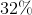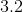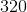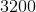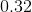Explanation:

In order to find the decimal equivalent for a percent, all we have to do is divide our percent by 100. We can also move the decimal point to the left two spaces.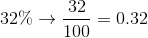### Example Question #19 : How To Find Decimal Equivalent To A Percentage

Find the decimal equivalent of the following percent: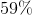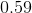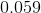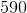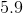Explanation:

In order to find the decimal equivalent for a percent, all we have to do is divide our percent by 100. We can also move the decimal point to the left two spaces.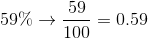### Example Question #20 : How To Find Decimal Equivalent To A Percentage

Find the decimal equivalent for the following percent: